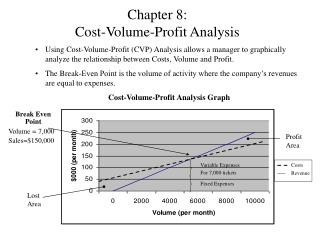Download PresentationChapter 8: Cost-Volume-Profit Analysis

# Chapter 8: Cost-Volume-Profit Analysis

Download Presentation## Chapter 8: Cost-Volume-Profit Analysis

- - - - - - - - - - - - - - - - - - - - - - - - - - - E N D - - - - - - - - - - - - - - - - - - - - - - - - - - -
##### Presentation Transcript

1. Chapter 8:Cost-Volume-Profit Analysis • Using Cost-Volume-Profit (CVP) Analysis allows a manager to graphically analyze the relationship between Costs, Volume and Profit. • The Break-Even Point is the volume of activity where the company’s revenues are equal to expenses. • Cost-Volume-Profit Analysis Graph Break Even Point Volume =7,000 Sales=\$150,000 Profit Area Variable Expenses For 7,000 tickets Costs Revenue Fixed Expenses Lost Area

2. CVP Analysis Equations Fixed expenses + Target net profit = Number of sales units required Unit contribution margin to earn target net profit Fixed expenses + Target net profit = Dollar sales required to earn Contribution margin ratio target net profit Budgeted sales revenue - break-even revenue = Safety margin Fixed expenses = Break-even point (in units) Unit contribution margin

3. Managerial Implications of ABC and CVP • Activity-based Costing (ABC) can provide a better description of a company’s cost behavior and CVP relationships. • An ABC CVP recognizes that some costs that are fixed with respect to sales volume may not be fixed with respect to other cost drivers. Make sure that the Bala’s Beer Company practice problem from course pack is included after this slide.